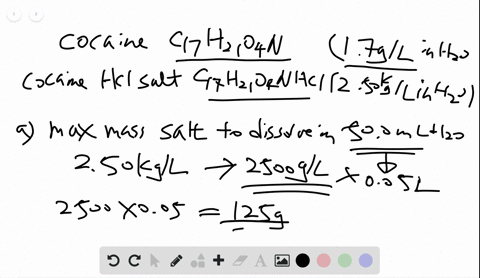Black Friday is Here! Start Your Numerade Subscription for 50% Off!Join Today## Discussion

You must be signed in to discuss.
Skyler H.
September 12, 2020
Skyler H.
September 12, 2020
I have worked out a problem with this video guide and the mass of SO2 is incorrect

## Video Transcript

given initial quantities, we can calculate the percent yield as well as the theoretical you. The first step is to be sure your equation is balance. So in this case you ate here and an eight here and we know the initial masses as well as the actual yield, it's sometimes helpful to start by converting two moles using the molar Mass. So we do that by taking our initial mass and dividing by the molar mass so that we have initially 1.27 moles of zinc and 0.204 moles. Oh, sulfur, and we see that we form one 0.7 moles of zinc silver. To find the limiting re agent. We take both of our initial reactions and see how much product should for so we do this by using the ratio from the balanced equation. So if I have 1.27 moles of zinc, Balanced Equation tells May that for every eight moles of sink, I should form eight moles of product or 1.27 rules zinc sulfide. If I have 0.204 moles of sulfur, I will form eight moles of product for every one mole, the silver or 1.6 three moles. I think sulfide, since 1.27 is less than 1.63 My living re agent is ink, so that means I should make 1.27 moles of zinc changing to grants using the molar mass of zinc sulphide, which is 97. I went for four. It should make 100 and 24.0 grams. This is called the Theoretical Yield. And so my percent yield is what I actually make, or 100 and 4.4 grams divided by my theoretical yield 1 20 1 24 grams times 100 or 84.2%. So my percent yield for this reaction is 84.2%. There are some additional side reactions that happened Does think can react with oxygen to make zinc oxide and sulfur can react with oxygen to make sulfur dioxide. And to predict how much of each of these products we need to look at, how much zinc remains based on how much we used to make the yield. So again. Initially, we had 1.27 malls of zinc and 0.204 Moles of Sulfur The change is how much it took to make my moles of product. And so if I had 1.7 bowls of zinc sulfide and my ratio is for every eight moles of product, I need eight moles of zinc. That means I would use up 1.7 So my remaining amount is 1.27 minus 1.7 or 0.20 Moles Inc. And similarly, my change in sulfur looks at how much zinc sulfide formed, knowing that for every eight moles of zinc sulfide, I need one mole of sulfur. So I've used up 0.13 moles. So for leaving me with the remaining amount of zero 0.74 moles. So these were the quantities that we can use to predict how much product away. If I have point two moles zinc, my balanced equation tells me I'll make two moles of zinc oxide for every two moles of zinc. And then I'm gonna multiply that by the more mass of zinc oxide which is 81 point 379 So this tells me that I should make 16.27 grams of zinc oxide as a side product. If I take my 0.74 Moles of sulfur and my balanced equation tells me for every one mole of sulfur we'll make one mole of sulfur dioxide, which Ingram's multiplying by the molar mass of 64 0.58 grams for every mole. I should make 4.74 grams of sulfur dioxide as a side product.